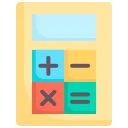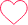# Tips and Tricks and Shortcuts for Circular Permutation Questions

## Tips and Tricks for Circular Permutations Questions

In this Page you will find out Tips, Tricks and Shortcuts for Solving Circular Permutation Questions. Apart from that you will get the important points that has to be remembered while solving the Circular Permutation based Questions.### Tips and Tricks for Circular Permutation

Circular Permutation are arrangements in the closed loops.

• If clockwise and anti-clock-wise orders are different, then total number of circular-permutations is given by (n-1)!
• If clock-wise and anti-clock-wise orders are taken as not different, then total number of circular-permutations is given by $\frac{(n-1)!}{2!}$
• While Solving Typical Problems divide Problems in Small Parts then think upon it.

### Points To Remember:

Here are some points to be kept in mind while solving the questions:

1. Choose a starting point: Even though the starting point doesn’t matter in circular arrangements, it can be helpful to pick a reference point to make the problem easier to solve.
2. Think linearly: Sometimes, it’s easier to pretend the objects are in a straight line instead of a circle. Treat the ends as neighbors, and then use regular counting methods for linear arrangements.
3. Treat groups together: If some objects must stay together or stay apart, treat them as one unit. This simplifies the problem and lets you consider arrangements of these groups and other individual objects separately.
4. Use (n-1)! for circles: For arranging n different objects in a circle, the number of ways is (n-1)!. This accounts for the circular arrangement and avoids overcounting.
5. Watch for repeated patterns: Rotations and reflections can create the same arrangements. Be careful and divide the total arrangements accordingly to avoid counting duplicates.
6. Handle rules step-by-step: If there are specific rules or constraints, apply them thoughtfully. Break down the problem into smaller parts to deal with different scenarios if needed.
7. Visualize the problem: Draw pictures or imagine the circular arrangement to better understand the situation. Visualization can help you identify patterns and decide on the best approach.
8. Practice with different situations: Circular permutation questions can vary, so practice with various scenarios to get better at solving them.

By using these tips and practicing regularly, you’ll get better at solving circular permutation problems without much difficulty.

### Prime Course Trailer

Get PrepInsta Prime & get Access to all 200+ courses offered by PrepInsta in One Subscription

### Type 1: When clockwise and anticlockwise arrangements are different.

• Trick: Number of circular permutations (arrangements) of n distinct things when arrangments are different = (n−1)!

Question 1. In how many ways can 5 girls be seated in a circular order?

Options:

1. 45
2. 24
3. 12
4. 120

Solution     Number of arrangements possible = (5 − 1)!

= 4!

= 4 x 3 x 2 x 1

= 24

Correct option: 2

Question 2 . Determine the number of ways in which 5 married couples are seated on a Circular Round table if the spouses sit opposite to one another.

Options

1. 120
2. 320
3. 384
4. 387

Solution   5 Married couples means we have to arrange 10 peoples

First women can be placed anywhere in a circular Round Table and her husband in 1 way.

Second women can be placed in 8 ways and her husband in 1 way.

Third woman can be placed in 6 ways and her husband in 1 way.

Fourth women can be placed in 4 ways and her husband in 1 way.

Fifth women can be placed in 2 ways and her husband in 1 way.

Total number of ways $1\times 8\times 1 \times 6\times 1 \times 4\times 1 \times 2 \times 1 = 384$Ways

Correct option: 3

### Type 2: When clockwise and anticlockwise arrangements are not different

• Tips & Trick: Number of circular permutations (arrangements) of n distinct things when arrangements are not different = $\frac{1}{2}$ × (n−1)!

Question 1. In how many ways can 8 beads can be arranged to form a necklace?

Options

1. 2520
2. 5040
3. 360
4. 1200

Solution     Since In formation of Necklace the Clockwise and Anti-clockwise Arrangements are Same So we divide by 2

Number of arrangements possible = $\frac{1}{2}$ × (n−1)!

$\frac{1}{2}$ × (8−1)!

$\frac{1}{2}$ × 7!

$\frac{1}{2}$ × 5040 = 2520

Correct option: 1

## Get over 200+ course One Subscription

Courses like AI/ML, Cloud Computing, Ethical Hacking, C, C++, Java, Python, DSA (All Languages), Competitive Coding (All Languages), TCS, Infosys, Wipro, Amazon, DBMS, SQL and others

## Checkout list of all the video courses in PrepInsta Prime Subscription

### One comment on “Tips and Tricks and Shortcuts for Circular Permutation Questions”

•rahulpeddipaga9177

thank you prepinsta creators for great minded thinking in this education system.0